# Chemical Equation Balance

4.5
5.21K reviews
500K+Everyone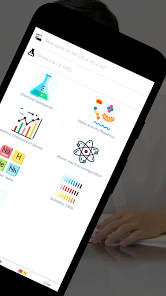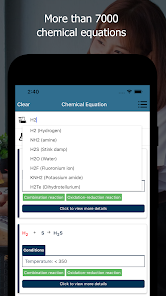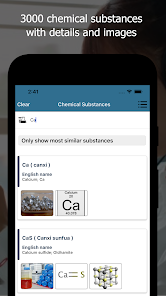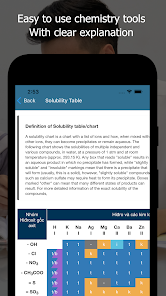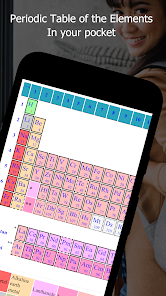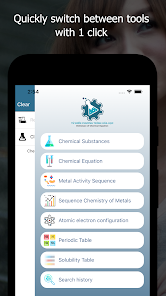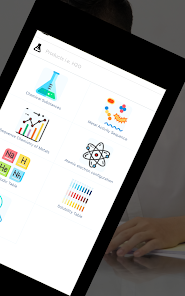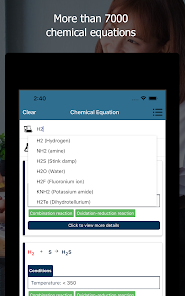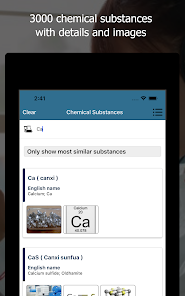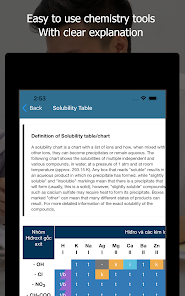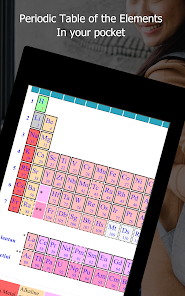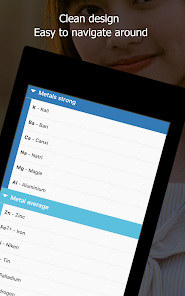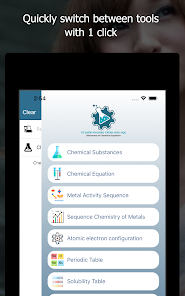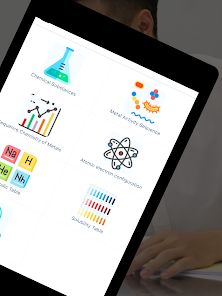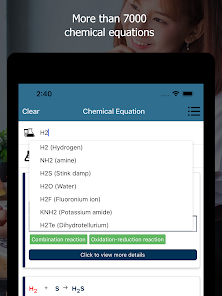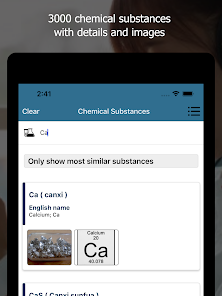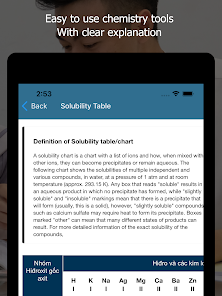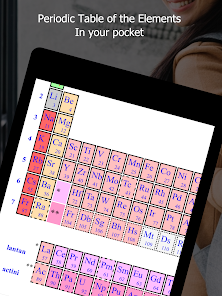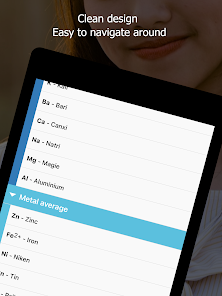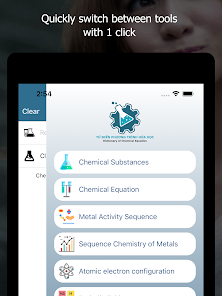++ Search with more than 7000 equations and 3000 chemicals ++
With many popular tools

NO CONNECTION TO INTERNET REQUIRED

Chemical equations solved with chemical equation balance app. This app specially designed for students for chemistry problems and work as equation solver, here you can search more than 7000 equations and 3000 chemicals. And in which you can balance equation within seconds, the equation shows the detail of substance's colour and form when participating chemical reaction. Chemical equation balance app doesn’t require internet connection. Chemical equation balance app is the best equation balancer app and also work as chem calculator or chemical balancer.

This chemical equation balance solve equation in seconds just write down the equation in given box then this chemical equation balance will solve equation and show the result with complete detail that is very helpful for you. Mostly student of colleges and universities face the problem in chemistry formulas and equations, now in this solving equations app you can resolve chemistry formulas and chemistry equations.

A chemical equation balance app not have only equation balance facility, it contains more information related to chemical. This app will balance chemical equations by providing you with the coefficients of the equation entered.

This chemical equation balancer app contains the:

1. Chemical Equation
2. Chemical Substances
3. Metal Activity
4. Periodic Table
5. Solubility Table

This app contains the equations solutions in it and also have a collection of data. If you have any doubt related to equations questions then you can put the equations in empty fields and get the balanced equation with the help of equation balancer. And the best part of this chemical equation balance app is it shows the complete detail that is very helpful to understand the equations.
Chemical equation balancer contains the chemical elements complete information in that equation balancer app. solving equation by hand is very difficult in minimum time and students face that issue during tests or papers or also when they are in hurry, due to this issue we developed this app for students for their easiness. Now they can only write the equation and find the solution. Because manual equation solving process is difficult and time taking now with the help of chemical equation balance app you can put equations and get the balanced equation with complete info that are helpful to explain any other person.

If you are not searching for chemical equation balancer then you can also found some extra knowledge and can get the information from this best ever chemical equation balance app. Because it contains the chemical elements, if you forgot about chemical elements then this app have this facility also you can see the chemical elements in the app. This app also contains the atomic configuration that helps to find by elemental name and it’s easy to find it out.

Features:

- Search with more than 7000 equations and 3000 chemicals
- The equation shows the detail of substance's colour and form when participating chemical reaction
- No connection to Internet required
- Many popular chemistry tools
- Limiting reagent calculations.
- Product mass-based calculations
- Get required reactant weights for desired products
- Instantly view details of elements. Balance chemical reactions and equations
- Find Molar Mass of Compounds

Chemical equation balance app is a learning app and also designed for students that contain the chemical equation, substances, and periodic table of elements. That also contains the basic chemical knowledge for a chemistry student and this chemical equation balance app also beneficial for a teacher also they can practice with chemistry equations and before lectures can prepare their lectures with the help of equation balance app. This is really helpful and best ever app in playstore.

Proudly developed in Vietnam
Updated on
Mar 27, 2022

## Data safetyNo information available
4.5
5.21K reviews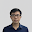Luong Duc Huy
April 18, 2021
As of writing this the app got an update, and after installing said update the app would just crash not even 15s into using it. It also breaks after you look up for literally one equation. Before it worked fine, but now it's a buggy mess. PLS FIX IT Edit: issue seems to have been fixed after the latest update. Works perfectly fine now
16 people found this review helpful
April 18, 2021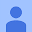April 11, 2021
I downloaded this app for help with understanding multi-step algebraic equations and how to solve them. I did not realize that the app would actually show me step-by-step how to solve problems! Each step is always detailed, and makes sense. Overall, I'm glad I downloaded this!!! Highly recommend.
7 people found this review helpful